Q. 1. What are electromagnetic waves ? Describe their properties. Describe electroma gnetic spectrum.

Ans ⇒ Electromagnetic waves : Such waves for their transmission no material medium is needed called electromagnetic waves.

Origin : We know that when current is allowed to flow through a long wire then a magnetic field is set up around it for infinite distance. This magnetic field is inversely proportional to distance.
Does this force field produced in total space due to current ? it is not so, because sudden production of magnetic fields can produce large electrical effect. So we guess that magnetic field expands far from wire with uniform velocity. When it happens so then variable magnetic field produces electric field. When this electric field travels with magnetic field then there is change in electric flow. Due to this electric flow magnetic field is generated. Due to this mutual interaction electric and magnetic fields are transmitted. Let B-field be travelling to the right with velocity v. We now consider the loop pqmn in XY plane.
Since plane has infinite expansion. So, B will be same at any distance.
The arm qm of loop is inside field, but pn is outside. Electric field E has been shown in fig. then, using Faraday’s law of electromagnetic induction we have
E x qm = Bv x qm or, E = Bv,                                     …(i)
Where u is velocity of electromagnetic wave.
Now we consider on a loop g hkl in xz/plane.
Then B x gl = μ0με0E x v x gl or, B = μ0ε0 Ev          …(ii)
.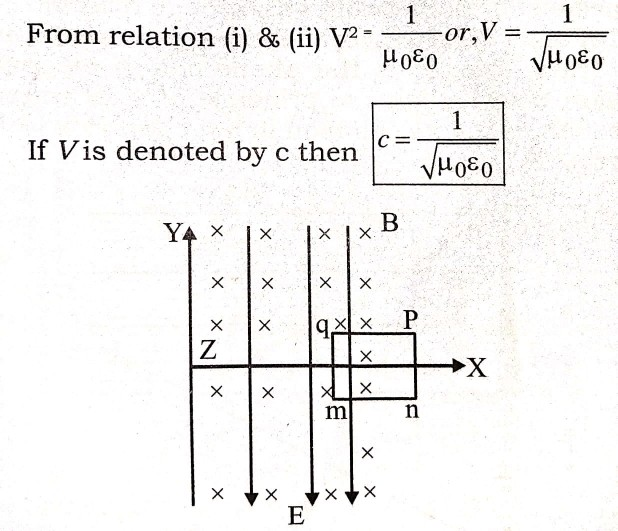Since μ0 = 4π x10-7, ε0 = 8.85 x 10-12 SI unit and C = 2.998 x 108 m/s ≃ 3 x 108 m/s
i.e, velocity of electromagnetic wave is equal to speed of light.
First of all, Hertz produce electro magnetic wave in 1889 and detected. Marconic used it in sending i message from one place to the other.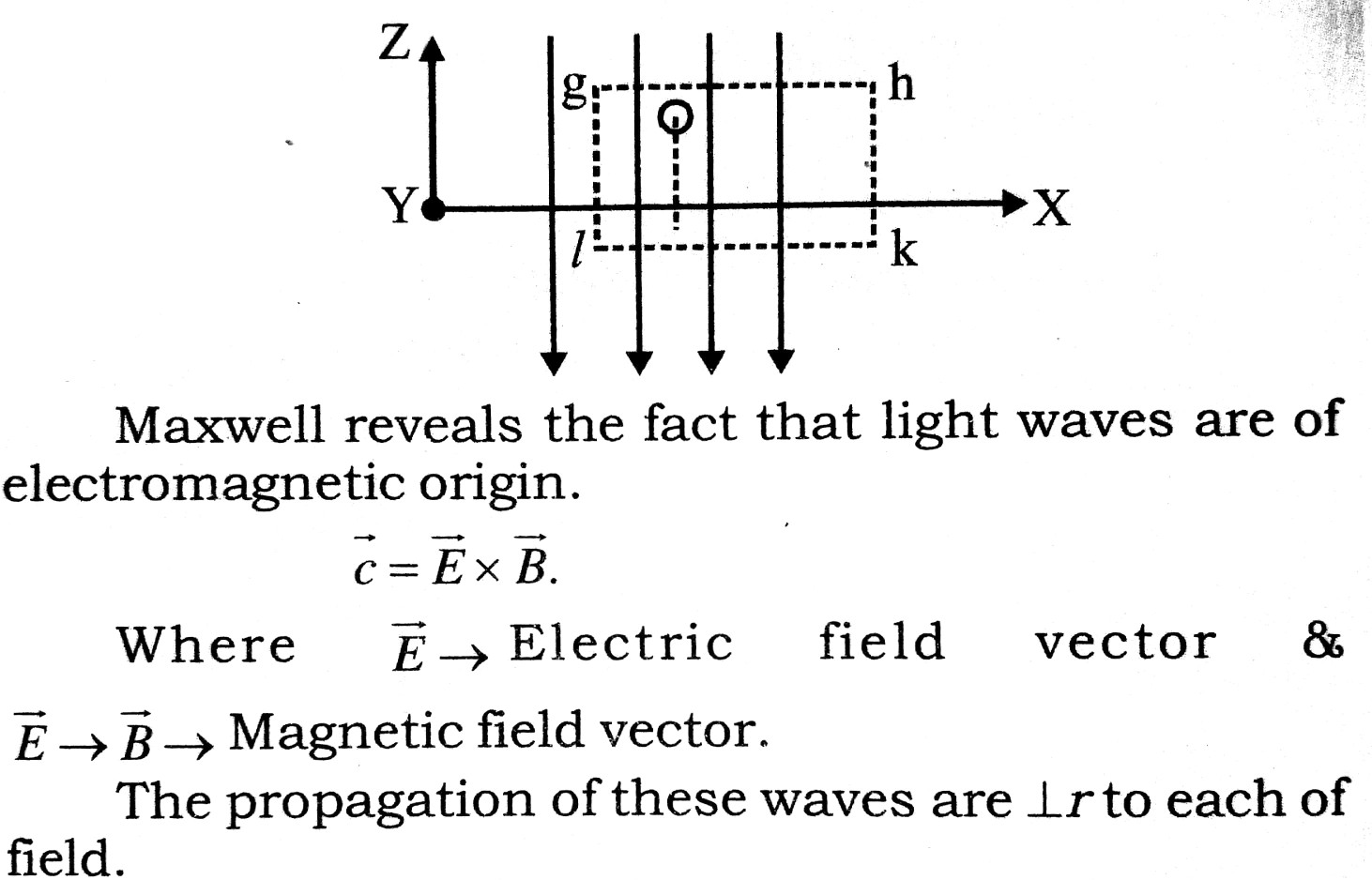Electromagnetic wave spectrum : Electromagnetic wave spectrum are folowing :

 Sl. No Name Frequency Wave length Uses 1. D.C. or Voltage 0 Hz ∞ D.C. machines, Transistors etc. 2. Powerline (50 – 60)Hz (6 x 103 – 5 x 103)Km A.C. machines, Industrial Power 3. Audible frequency 20 Hz -20 KHz (15 x 103 – 15) km Micro phone, Loudspeaker 4. Ultrasonic or very low radio frequencies (15 – 30)KHz (20 – 10 Km) For cleaning or research work 5. Radio waves 30Khz – 30MHz (10km – 10)m Radio communication 6. High frequency radio waves 30 – 300 MHz (10 – 1)m FM & TV communication 7. Ultra high radio waves. (300 -3000)MHz 1m – 10cm TV radar 8. Microwaves (1 – 300)GHz 30 cm – 1mm Satellite communication etc. 9. Heat radiation (3 x 109 – 3 x 1013) (10 x 10cm – mm)

Q. 2. What do you mean by displacement current ? How would you express Maxwell-Ampere Law using it ?

Ans ⇒ According to Ampere’s law, the line lintegral of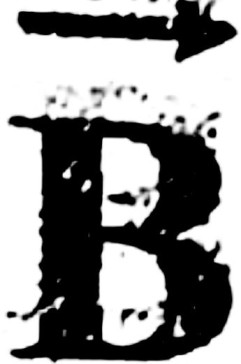is proportional to the algebraic sum of currents crossing the surface bounded by the integration path.
.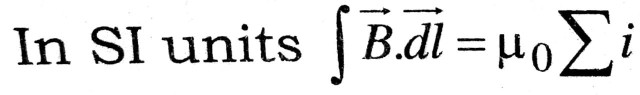When this law is applied to a parallel capacitor plate being charged, the law gets a question mark. In figure, the law is valid for S1.
.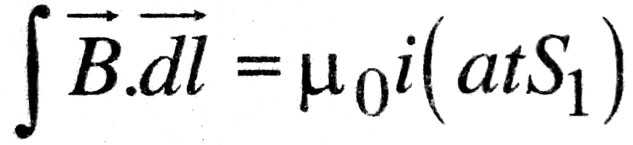But at S2 the law fails an no charge is crossing S2, i = 0. then
.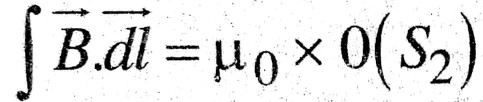The linear integral is different. Maxwell removed the difficulty by defining displacement current. We have q = CV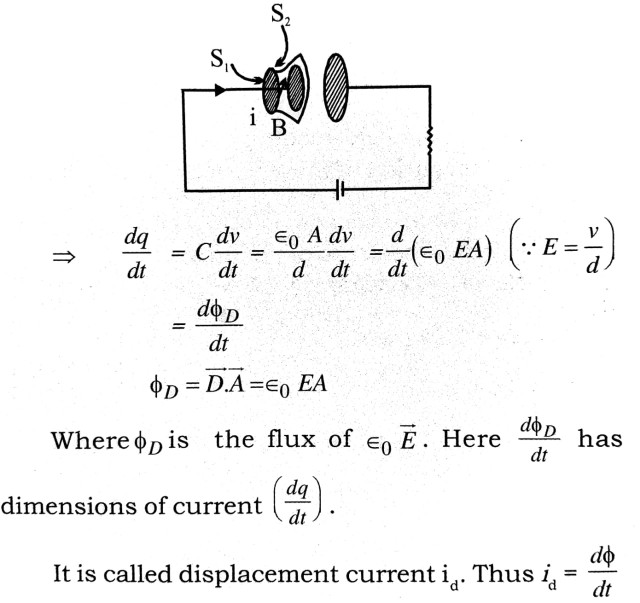It is not due to flow of current but due to rate of flux change. It has no thermal effect but it shown magnetic effect.
Maxwell-Ampere Law : The line integral of magnetic field over a closed loop is proportional to the algebraic sum of conduction current (id). In SI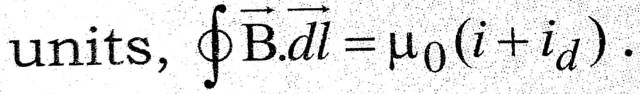## Class 12th physics Long Type question in English

 S.N Physics Long Type Question English Medium 1. ELECTRIC CHARGES AND FIELDS 2. LECTROSTATIC POTENTIAL AND CAPACITANCE 3. 4. 5. MAGNETISM AND MATTER 6. ELECTROMAGNETIC INDUCTION 7. ALTERNATING CURRENT 8. ELECTROMAGNETIC WAVES 9. RAY OPTICS AND OPTICAL INSTRUMENTS 10. WAVE OPTICS 11. DUAL NATURE OF MATTER AND RADIATION 12. ATOMS 13. NUCLEI 14. SEMI CONDUCTOR ELECTRONICS 15. COMMUNICATION SYSTEMS
You might also like